C++ Programs to Calculate Sum Of Digits

In this tutorial you will learn about the C++ Programs to Calculate Sum Of Digits and its application with practical example.

C++ Program to Print Sum of Digits

In this tutorial, we will learn to create a C++ program that will Print Sum of digits in a given Number using C++ programming.

Prerequisites

Before starting with this tutorial we assume that you are best aware of the following C++ programming topics:

• Operators in C++ Programming.
• Basic Input and Output function in C++ Programming.
• Basic C++ programming.
• While loop in C++ programming.
• Conditional Statements in C++ programming.
• Arithmetic operations in C++ Programming.

Program to Print Sum of digits in a given Number

In c++ programming, we will make a program in today’s tutorial. We will take integer input from the user and find Sum of digits in a given number with the help of a program.

With the help of this program, we can Print Sum of digits in a given number.

Output:-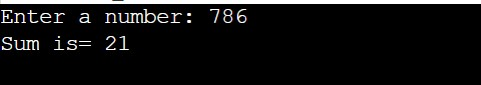In the above program, we have first initialized the required variable.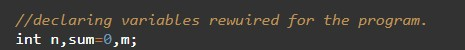• n = it will hold the integer value of the input.
• sum = it will hold the integer value for sum.
• m = it will hold the integer value.

Input number from the user.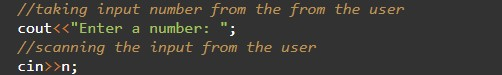Program Logic Code.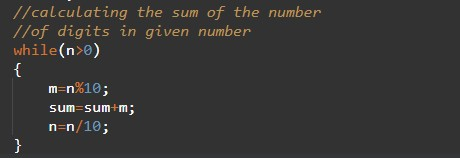Printing output for sum the digits.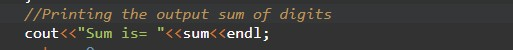In this tutorial we have learn about the C++ Programs to Calculate Sum Of Digits and its application with practical example. I hope you will like this tutorial.